/

### The Poisson distribution

The Poisson distribution expresses the probability of a given number of events occurring in a fixed interval of time if the events occur with a known average rate and independently of the time since the last event.

 Distribution name Poisson distribution Common notation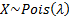Parameters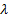= event rate (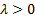) Support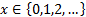Probability mass function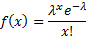Cumulative distribution function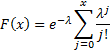(can also be expressed using the incomplete gamma function) MeanVarianceSkewness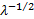(Excess) kurtosis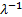Characteristic function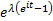Other comments The median is approximately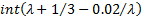.   The mode is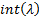ifis not integral. Otherwise the distribution is bi-modal with modesand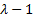.

Nematrian web functions

Functions relating to the above distribution may be accessed via the Nematrian web function library by using a DistributionName of “poisson”. For details of other supported probability distributions see here.